# Division + subtraction - math problems

#### Number of problems found: 38

• What is 13What is the number sentence? * 1 point 1/2 + 3/4 + 1/4 = A 1/2 - 3/4 - 1/4 = B 1/2 x 3/4 x 1/4 = C 1/2 ÷ 3/4 ÷ 1/4 = D
• Milka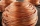Milka has three and seven sixteeths cm of wire. She needs only two and five eighths cm of wire for her project in the laboratory. How much wire does she need to cut?
• Benhur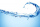Benhur boiled 1 1/4 liters of water in a kettle. After 10 1/2 minutes he measured the water again. It had 3/4 liters left in the kettle. What is the amount of water that evaporates every minutes?
• Jose studiedJose studied for 4 and 1/2 hours on Saturday and another 6 and 1/4 hours on Sunday. How many subjects did he study if he has alloted 1 and 1/2 hours per subject on Saturday and 1 and 1/4 hours per subject on Sunday?
• Nice two numbersWhat two numbers we give a sum of 30 difference of 24 products of 81 and a quotient of 9?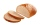Fahima buys 2 packets of bread rolls costing £1.50 for each packet 1 bottle of ketchup costing £1.60 3 packets of sausages Fahima pays with a £10 note. She gets 30p change. Fahima works out that one packet of sausages costs £2.30. Is Fahima right?
• Frame image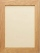Peter wants to put a picture in a frame. The picture is 7 3/5 cm wide. To fit in the picture frame cannot be more than 7 3/10 cm wide. How much should the image be trimmed?
• Simplify expression with mixedWhen (7¹/3 + 2¾)÷(2 + 2¼ × 1¹/3) is simplified the result is?
• Fraction expressionWhich expression is equivalent to : minus 9 minus left parenthesis minus 4 start fraction 1 divided by 3 end fraction right parenthesis
• ItemsItems Acosts 675.35 while item B costs 1605.25. by how much more was the cost of item B than item A. If item A and B will be purchased, how much would are pay?
• Jolly gobs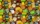Each package of jolly gobs has 72 gobs. If one fourth of the gobs are red and the rest are blue, into how many parts was the group divided? How many parts are red?
• Evaluate valueEvaluate value of the following expressions: 1. The product of nine and a number 12, decreased by six 2. Eight decreased by three times a number 2. 3. The quotient of twice a number 49 and seven.
• Fractions and mixed numerals(a) Convert the following mixed numbers to improper fractions. i. 3 5/8 ii. 7 7/6 (b) Convert the following improper fraction to a mixed number. i. 13/4 ii. 78/5 (c) Simplify these fractions to their lowest terms. i. 36/42 ii. 27/45 2. evaluate the follow
• If the 3If the temperature of a city drops 2° everyday and increases by 3° on every 5 days, how long does it take to drop to -15° from temperature +5°?
• Metal rodYou have a metal rod that’s 51/64 inches long. The rod needs to be trimmed. You cut 1/64 inches from one end and 1/32 inches from the other end. Next, you cut the rod into 6 equal pieces. What will be the final length of each piece?
• How manyHow many integers are greater than 547/3 and less than 931/4?
• Six-eightsSix-eights of the one hundred pupils joined the Math Glee club. If the Math Glee club members were grouped into three, how many members were in each group?
• Trip cost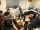In September, the trip cost CZK 12,000. How many crowns did the trip cost in June of the same year, when they have since reduced the price by a quarter and by another CZK 1,200?
• A farmer's heritage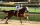A farmer died leaving his 17 horses to his 3 sons. When his sons opened up the Will it read: My eldest son should get 1/2 (half) of total horses; My middle son should be given 1/3rd (one-third) of the total horses; My youngest son should be given 1/9th (o
• In the bowlThere are 12 pears in the bowl. Erik ate a third. How many pears remained in the bowl?

Do you have an exciting math question or word problem that you can't solve? Ask a question or post a math problem, and we can try to solve it.

We will send a solution to your e-mail address. Solved examples are also published here. Please enter the e-mail correctly and check whether you don't have a full mailbox.

Division Problems. Subtraction Problems.